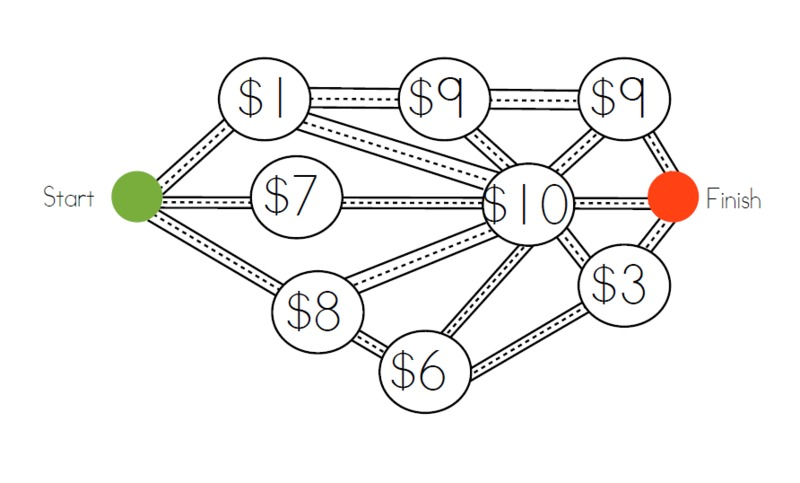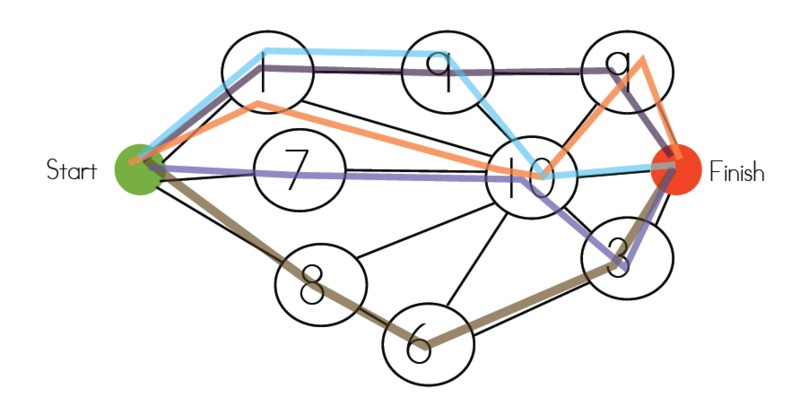# \$20 Dot Map Alignments to Content Standards: 1.OA.C.6 ## Task The attached graphic shows a map. You must get from start to finish by visiting three of the dots, at each dot you have to pay the specified number of dollars. If you have \$20 can you get from start to finish and visit three dots?Bonus Question #1: Can you find a way to get from start to finish and spend all \$20? Can you find a way to get from start to finish and spend less then \$20?

Bonus Question #2: How many different routes can you find from start to finish that go to three dots and cost \\$20 or less?

## IM Commentary

The language for this task is written above a 1st grade reading level, so it will need to be introduced verbally by the teacher. This problem helps students to practice adding three numbers whose sum are 20 or less. It is an open-ended problem with many solutions. This problem would work well either as a whole group or students could work on it as individuals or pairs. If this problem is presented whole group, the teacher needs to blow the graphic up using an Elmo, Smart Board or overhead projector. If students are going to work on it in pairs they will need to be given print-outs of the graphic.

## Solution

$$1 + 9 + 9 = 19$$

$$1 + 9 + 10 = 20$$

$$1+ 10 + 9 = 20$$ $$7 + 10 + 3 = 20$$$$8 + 6 + 3 = 17$$$$1+10+3=14$$